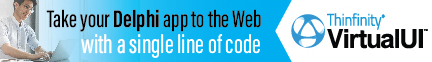DelphiBasicsSqrFunction Gives the square of a number System unit
 1 function Sqr ( Number :  Integer ) : Integer; 2 function Sqr ( Number :    Int64 ) : Int64; 3 function Sqr ( Number : Extended ) : Extended;
Description
The Sqr function returns the square of a Number.

Sqr(Number) = Number * Number

The number may be an integer or floating point type.

Integer, Int64 numbers

If the square of the number exceeds the capacity of the target variable, then the result is :

Result Mod Capacity

Extended numbers

If the square of the number exceeds the capacity, EOverFlow exception is raised.

Special values are treated as follows:

 Infinity, -Infinity : Infinity NaN (Not a Number) : NaN
Related commands
 Dec Decrement an ordinal variable Inc Increment an ordinal variable Sqrt Gives the square root of a number Sum Return the sum of an array of floating point valuesDownload this web site as a Windows program.